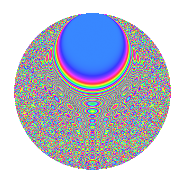# Properties

 Label 3150.2.crLevel 3150 Weight 2 Character orbit cr Rep. character $$\chi_{3150}(361,\cdot)$$ Character field $$\Q(\zeta_{15})$$ Dimension 800 Sturm bound 1440

# Learn more about

## Defining parameters

 Level: $$N$$ = $$3150 = 2 \cdot 3^{2} \cdot 5^{2} \cdot 7$$ Weight: $$k$$ = $$2$$ Character orbit: $$[\chi]$$ = 3150.cr (of order $$15$$ and degree $$8$$) Character conductor: $$\operatorname{cond}(\chi)$$ = $$175$$ Character field: $$\Q(\zeta_{15})$$ Sturm bound: $$1440$$

## Dimensions

The following table gives the dimensions of various subspaces of $$M_{2}(3150, [\chi])$$.

Total New Old
Modular forms 5888 800 5088
Cusp forms 5632 800 4832
Eisenstein series 256 0 256

## Trace form

 $$800q$$ $$\mathstrut +\mathstrut 100q^{4}$$ $$\mathstrut -\mathstrut 2q^{5}$$ $$\mathstrut +\mathstrut O(q^{10})$$ $$800q$$ $$\mathstrut +\mathstrut 100q^{4}$$ $$\mathstrut -\mathstrut 2q^{5}$$ $$\mathstrut +\mathstrut 2q^{10}$$ $$\mathstrut +\mathstrut 6q^{11}$$ $$\mathstrut +\mathstrut 100q^{16}$$ $$\mathstrut +\mathstrut 4q^{19}$$ $$\mathstrut +\mathstrut 4q^{20}$$ $$\mathstrut -\mathstrut 32q^{22}$$ $$\mathstrut -\mathstrut 14q^{23}$$ $$\mathstrut +\mathstrut 48q^{26}$$ $$\mathstrut -\mathstrut 2q^{28}$$ $$\mathstrut +\mathstrut 24q^{29}$$ $$\mathstrut -\mathstrut 6q^{31}$$ $$\mathstrut -\mathstrut 16q^{34}$$ $$\mathstrut -\mathstrut 24q^{35}$$ $$\mathstrut -\mathstrut 20q^{37}$$ $$\mathstrut -\mathstrut 8q^{38}$$ $$\mathstrut +\mathstrut 2q^{40}$$ $$\mathstrut -\mathstrut 4q^{41}$$ $$\mathstrut -\mathstrut 72q^{43}$$ $$\mathstrut -\mathstrut 4q^{44}$$ $$\mathstrut +\mathstrut 12q^{46}$$ $$\mathstrut +\mathstrut 20q^{47}$$ $$\mathstrut -\mathstrut 16q^{50}$$ $$\mathstrut -\mathstrut 16q^{53}$$ $$\mathstrut +\mathstrut 24q^{55}$$ $$\mathstrut +\mathstrut 8q^{58}$$ $$\mathstrut +\mathstrut 8q^{61}$$ $$\mathstrut -\mathstrut 200q^{64}$$ $$\mathstrut +\mathstrut 18q^{65}$$ $$\mathstrut +\mathstrut 32q^{67}$$ $$\mathstrut -\mathstrut 40q^{68}$$ $$\mathstrut +\mathstrut 46q^{70}$$ $$\mathstrut +\mathstrut 4q^{71}$$ $$\mathstrut -\mathstrut 36q^{73}$$ $$\mathstrut +\mathstrut 16q^{74}$$ $$\mathstrut +\mathstrut 32q^{76}$$ $$\mathstrut -\mathstrut 8q^{77}$$ $$\mathstrut -\mathstrut 2q^{80}$$ $$\mathstrut -\mathstrut 64q^{82}$$ $$\mathstrut +\mathstrut 40q^{83}$$ $$\mathstrut +\mathstrut 12q^{85}$$ $$\mathstrut -\mathstrut 12q^{86}$$ $$\mathstrut -\mathstrut 14q^{88}$$ $$\mathstrut -\mathstrut 54q^{89}$$ $$\mathstrut -\mathstrut 4q^{91}$$ $$\mathstrut -\mathstrut 32q^{92}$$ $$\mathstrut +\mathstrut 32q^{94}$$ $$\mathstrut -\mathstrut 32q^{95}$$ $$\mathstrut +\mathstrut 132q^{97}$$ $$\mathstrut +\mathstrut 32q^{98}$$ $$\mathstrut +\mathstrut O(q^{100})$$

## Decomposition of $$S_{2}^{\mathrm{new}}(3150, [\chi])$$ into irreducible Hecke orbits

The newforms in this space have not yet been added to the LMFDB.

## Decomposition of $$S_{2}^{\mathrm{old}}(3150, [\chi])$$ into lower level spaces

$$S_{2}^{\mathrm{old}}(3150, [\chi]) \cong$$ $$S_{2}^{\mathrm{new}}(175, [\chi])$$$$^{\oplus 6}$$$$\oplus$$$$S_{2}^{\mathrm{new}}(350, [\chi])$$$$^{\oplus 3}$$$$\oplus$$$$S_{2}^{\mathrm{new}}(525, [\chi])$$$$^{\oplus 4}$$$$\oplus$$$$S_{2}^{\mathrm{new}}(1050, [\chi])$$$$^{\oplus 2}$$$$\oplus$$$$S_{2}^{\mathrm{new}}(1575, [\chi])$$$$^{\oplus 2}$$